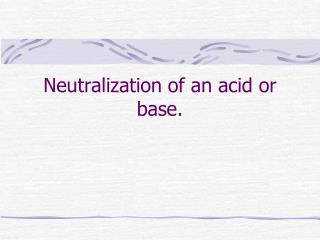DownloadDownload PresentationNeutralization of an acid or base.

# Neutralization of an acid or base.

Télécharger la présentation## Neutralization of an acid or base.

- - - - - - - - - - - - - - - - - - - - - - - - - - - E N D - - - - - - - - - - - - - - - - - - - - - - - - - - -
##### Presentation Transcript

1. Neutralization of an acid or base.

2. Mixing acids and bases • ~creates water • H3O+ + OH- 2 H2O • this is called neutralizing the solution • a neutralized solution is no longer dangerous. • The point where neutralization is complete is called the equivalence point

3. Salts • ~the byproduct of an acid and a base. • NaOH + HCl  H2O + NaCl • (base) (acid) (water) (salt) • there are several more than just table salt. • HNO3 + NH4OH  H2O + NH4NO3 • Acid Base water salt

4. Gases can be created • this depends on the reactants (not all will) • sodium bicarbonate (baking soda) will pretty much always release a gas • NaHCO3 + H2SO4H2O + NaHSO4 +CO2 Salt Gas

5. Titration • ~mixing an acid and base perfectly to make a neutral solution. • You normally need some kind of indicator for this. • Phenolphthalein- when in solution turns red if basic and is clear if acidic. • You can also use a pH probe

6. Graph of titration equivalence point pH Volume strong base added

7. Using math • To neutralize a solution you will need to add an equal amount of H3O+ / OH- to what was already present. • so that • mol H3O+ = mol OH- • This is used if and only if you are at the equivalence point (completely neutral solution)!

8. Problem • If 94 mL of 4.0 M NaOH neutralizes 6.0 L of an unknown strong acid, what was the H3O+concentration of the unknown? • 4 M NaOH x .094 L = .376 mol NaOH • .376 mol H3O+/ 6.0 L = .063 M H3O+

9. Another problem • If 127 mL of 2.0 M NaOH neutralizes 4.1 L of an unknown acid, what is the initial concentration of the acid? • 2.0M(.127 L) = .254 mol NaOH • =.254 mol OH- = .254 mol H3O+ • 4.1 L • =.062 M